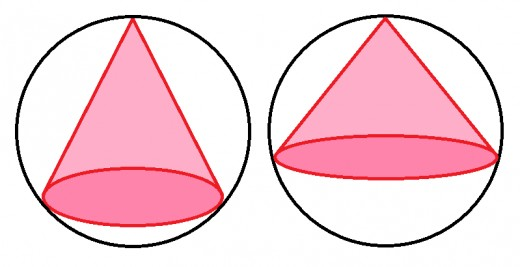# Cone you sphere the time to solve this?If the largest possible volume of a cone inscribed in a sphere of unit volume can be represented as $\frac{a}{b}$, where $a$ and $b$ are coprime natural numbers, what is the value of $a+b$?

×